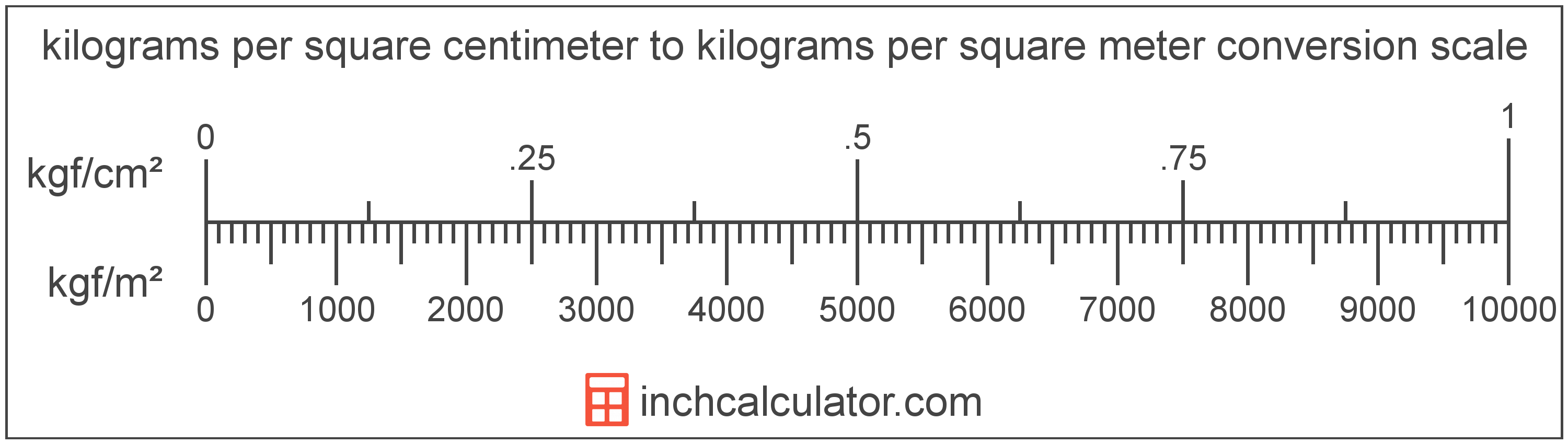# Kilograms per Square Centimeter to Kilograms per Square Meter Converter

Enter the pressure in kilograms per square centimeter below to get the value converted to kilograms per square meter.

Results in Kilograms per Square Meter:1 kgf/cm² = 10,000 kgf/m²

## How to Convert Kilograms per Square Centimeter to Kilograms per Square Meter

To convert a measurement in kilograms per square centimeter to a measurement in kilograms per square meter, multiply the pressure by the following conversion ratio: 10,000 kilograms per square meter/kilogram per square centimeter.

Since one kilogram per square centimeter is equal to 10,000 kilograms per square meter, you can use this simple formula to convert:

kilograms per square meter = kilograms per square centimeter × 10,000

The pressure in kilograms per square meter is equal to the pressure in kilograms per square centimeter multiplied by 10,000.

For example, here's how to convert 5 kilograms per square centimeter to kilograms per square meter using the formula above.
kilograms per square meter = (5 kgf/cm² × 10,000) = 50,000 kgf/m²Kilograms per square centimeter and kilograms per square meter are both units used to measure pressure. Keep reading to learn more about each unit of measure.

## What Are Kilograms per Square Centimeter?

One kilogram per square centimeter is the pressure of equal to one kilogram-force per square centimeter.

The kilogram per square centimeter is a non-SI metric unit for pressure. A kilogram per square centimeter is sometimes also referred to as a kilogram per square centimetre or kilogram-force per centimeter square. Kilograms per square centimeter can be abbreviated as kgf/cm²; for example, 1 kilogram per square centimeter can be written as 1 kgf/cm².

In the expressions of units, the slash, or solidus (/), is used to express a change in one or more units relative to a change in one or more other units. For example, kgf/cm² is expressing a change in weight relative to a change in area.

The unit is deprecated and not permitted for use with SI units, although it is still in use as some precision measuring devices still use the unit.

Kilograms per square centimeter can be expressed using the formula:
1 kgf/cm2 = 1 kgf / cm2

Pressure in kilograms per square centimeter are equal to the kilogram-force divided by the area in square centimeters.

## What Are Kilograms per Square Meter?

One kilogram per square meter is the pressure of equal to one kilogram-force per square meter.

The kilogram per square meter is a non-SI metric unit for pressure. A kilogram per square meter is sometimes also referred to as a kilogram per square metre or kilogram-force per meter square. Kilograms per square meter can be abbreviated as kgf/m²; for example, 1 kilogram per square meter can be written as 1 kgf/m².

The unit is deprecated and not permitted for use with SI units.

Kilograms per square meter can be expressed using the formula:
1 kgf/m2 = 1 kgf / m2

Pressure in kilograms per square meter are equal to the kilogram-force divided by the area in square meters.

## Kilogram per Square Centimeter to Kilogram per Square Meter Conversion Table

Table showing various kilogram per square centimeter measurements converted to kilograms per square meter.
Kilograms Per Square Centimeter Kilograms Per Square Meter
0.0001 kgf/cm² 1 kgf/m²
0.0002 kgf/cm² 2 kgf/m²
0.0003 kgf/cm² 3 kgf/m²
0.0004 kgf/cm² 4 kgf/m²
0.0005 kgf/cm² 5 kgf/m²
0.0006 kgf/cm² 6 kgf/m²
0.0007 kgf/cm² 7 kgf/m²
0.0008 kgf/cm² 8 kgf/m²
0.0009 kgf/cm² 9 kgf/m²
0.001 kgf/cm² 10 kgf/m²
0.002 kgf/cm² 20 kgf/m²
0.003 kgf/cm² 30 kgf/m²
0.004 kgf/cm² 40 kgf/m²
0.005 kgf/cm² 50 kgf/m²
0.006 kgf/cm² 60 kgf/m²
0.007 kgf/cm² 70 kgf/m²
0.008 kgf/cm² 80 kgf/m²
0.009 kgf/cm² 90 kgf/m²
0.01 kgf/cm² 100 kgf/m²
0.02 kgf/cm² 200 kgf/m²
0.03 kgf/cm² 300 kgf/m²
0.04 kgf/cm² 400 kgf/m²
0.05 kgf/cm² 500 kgf/m²
0.06 kgf/cm² 600 kgf/m²
0.07 kgf/cm² 700 kgf/m²
0.08 kgf/cm² 800 kgf/m²
0.09 kgf/cm² 900 kgf/m²
0.1 kgf/cm² 1,000 kgf/m²
0.2 kgf/cm² 2,000 kgf/m²
0.3 kgf/cm² 3,000 kgf/m²
0.4 kgf/cm² 4,000 kgf/m²
0.5 kgf/cm² 5,000 kgf/m²
0.6 kgf/cm² 6,000 kgf/m²
0.7 kgf/cm² 7,000 kgf/m²
0.8 kgf/cm² 8,000 kgf/m²
0.9 kgf/cm² 9,000 kgf/m²
1 kgf/cm² 10,000 kgf/m²

## References

1. National Institute of Standards and Technology, NIST Guide to the SI, Chapter 6: Rules and Style Conventions for Printing and Using Units, https://www.nist.gov/pml/special-publication-811/nist-guide-si-chapter-6-rules-and-style-conventions-printing-and-using